# SAT Math Multiple Choice Question 484: Answer and Explanation

### Test Information

Question: 484

4.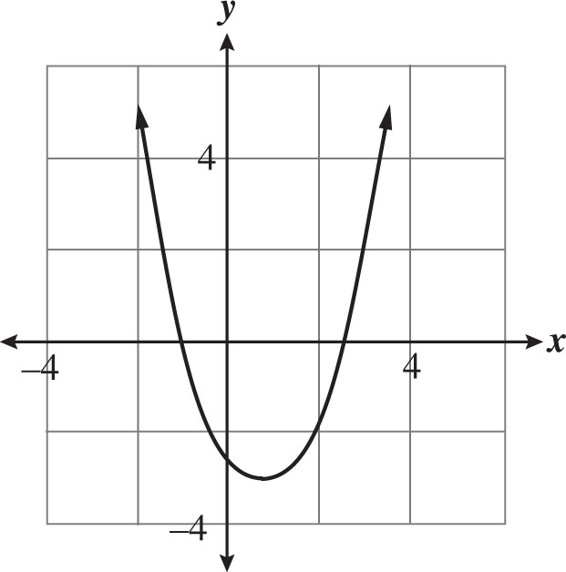Each of the following quadratic equations represents the graph shown above. Which equation reveals the exact values of the x-intercepts of the graph?

• A.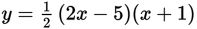• B.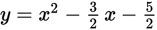• C.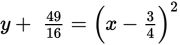• D.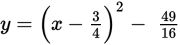Getting to the Answer: The factored form of a quadratic equation reveals the solutions to the equation, which graphically represent the x-intercepts. Choice (A) is the only equation written in this form and therefore must be correct. You can set each factor equal to 0 and solve to find that the x-intercepts of the graph are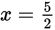and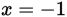.